# 图像处理做分量颜色直方图

## opencv提取图像中的颜色直方图（RGB、HSV）

2018-10-31 17:15:20 Jaster_wisdom 阅读数 10503
• ###### 利用图像分割和融合技术实现图片滤镜特效

通过本课程学习，初学者可以熟练使用opencv4 API完成各种常见的图像分割、识别任务。 中高级学习者也一定能有新的体会和收获。 本课程所讲的例子代码来自于实际开发项目，有较高的实用性。

20人学习 刘山
免费试看

#include "stdafx.h"
#include <iostream>
#include <opencv2/opencv.hpp>
using namespace cv;
using namespace std;

int main()
{
//读取本地的一张图片
imshow("原图", srcimage);
int channels = 0;
int histsize[] = { 256 };
float midranges[] = { 0,255 };
const float *ranges[] = { midranges };
MatND  dsthist;    //要输出的直方图
//重点关注calcHist函数，即为计算直方图的函数
calcHist(&srcimage, 1, &channels, Mat(), dsthist, 1, histsize, ranges, true, false);

Mat b_drawImage = Mat::zeros(Size(256, 256), CV_8UC3);
double g_dhistmaxvalue;
minMaxLoc(dsthist, 0, &g_dhistmaxvalue, 0, 0);
for (int i = 0;i < 256;i++) {
//这里的dsthist.at<float>(i)就是每个bins对应的纵轴的高度
int value = cvRound(256 * 0.9 *(dsthist.at<float>(i) / g_dhistmaxvalue));
line(b_drawImage, Point(i, b_drawImage.rows - 1), Point(i, b_drawImage.rows - 1 - value), Scalar(255, 0, 0));
}
imshow("B通道直方图", b_drawImage);

channels = 1;
calcHist(&srcimage, 1, &channels, Mat(), dsthist, 1, histsize, ranges, true, false);
Mat g_drawImage = Mat::zeros(Size(256, 256), CV_8UC3);
for (int i = 0;i < 256;i++) {
int value = cvRound(256 * 0.9 *(dsthist.at<float>(i) / g_dhistmaxvalue));
line(g_drawImage, Point(i, g_drawImage.rows - 1), Point(i, g_drawImage.rows - 1 - value), Scalar(0, 255, 0));
}
imshow("G通道直方图", g_drawImage);

channels = 2;
calcHist(&srcimage, 1, &channels, Mat(), dsthist, 1, histsize, ranges, true, false);
Mat r_drawImage = Mat::zeros(Size(256, 256), CV_8UC3);
for (int i = 0;i < 256;i++) {
int value = cvRound(256 * 0.9 *(dsthist.at<float>(i) / g_dhistmaxvalue));
line(r_drawImage, Point(i, r_drawImage.rows - 1), Point(i, r_drawImage.rows - 1 - value), Scalar(0, 0, 255));
}
imshow("R通道直方图", r_drawImage);

imshow("RGB直方图", r_drawImage);
waitKey(0);
return 0;
}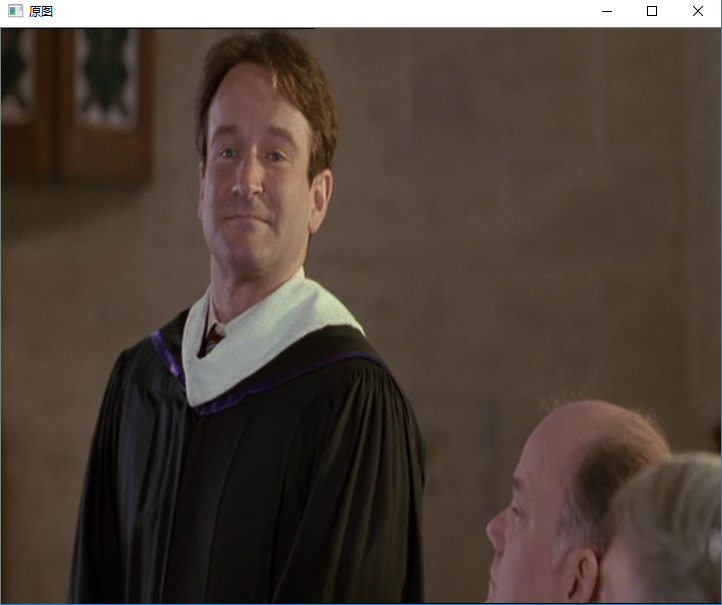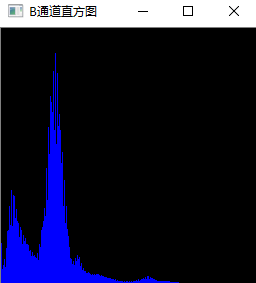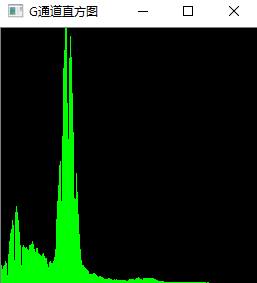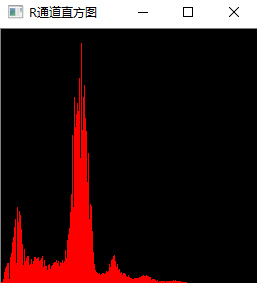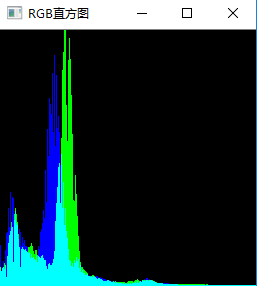HSV是一种将RGB色彩空间中的点在倒圆锥体中的表示方法。HSV即色相（Hue）、饱和度（Saturation）、亮度（Value），色相即颜色的基本属性，饱和度指色彩的纯度，越高则色彩越纯，亮度指色彩的明亮程度。

HSV颜色空间规定的取值范围： H：0~360，S：0~1，V：0~1

HSV颜色空间特征的提取方法和RGB类似，关键一点就是要将原图像转化为HSV颜色空间的图像，之后再对三个通道分别进行直方图绘制操作即可。

#include "stdafx.h"
#include <iostream>
#include <opencv2/opencv.hpp>
using namespace cv;
using namespace std;

int main()
{
imshow("原图", srcimage);
Mat srcimageHSV;
//图像转化HSV颜色空间图像
cvtColor(srcimage, srcimageHSV, COLOR_BGR2HSV);
imshow("HSV空间图像", srcimageHSV);
int channels = 0;
int histsize[] = { 256 };
float midranges[] = { 0,255 };
const float *ranges[] = { midranges };
MatND  dsthist;
calcHist(&srcimageHSV, 1, &channels, Mat(), dsthist, 1, histsize, ranges, true, false);
Mat b_drawImage = Mat::zeros(Size(256, 256), CV_8UC3);

double g_dhistmaxvalue;
minMaxLoc(dsthist, 0, &g_dhistmaxvalue, 0, 0);
for (int i = 0;i < 256;i++) {
int value = cvRound(256 * 0.9 *(dsthist.at<float>(i) / g_dhistmaxvalue));
line(b_drawImage, Point(i, b_drawImage.rows - 1), Point(i, b_drawImage.rows - 1 - value), Scalar(255, 0, 0));
}
imshow("H通道直方图", b_drawImage);

channels = 1;
calcHist(&srcimageHSV, 1, &channels, Mat(), dsthist, 1, histsize, ranges, true, false);
Mat g_drawImage = Mat::zeros(Size(256, 256), CV_8UC3);
for (int i = 0;i < 256;i++) {
int value = cvRound(256 * 0.9 *(dsthist.at<float>(i) / g_dhistmaxvalue));
line(g_drawImage, Point(i, g_drawImage.rows - 1), Point(i, g_drawImage.rows - 1 - value), Scalar(0, 255, 0));
}
imshow("S通道直方图", g_drawImage);

channels = 2;
calcHist(&srcimageHSV, 1, &channels, Mat(), dsthist, 1, histsize, ranges, true, false);
Mat r_drawImage = Mat::zeros(Size(256, 256), CV_8UC3);
for (int i = 0;i < 256;i++) {
int value = cvRound(256 * 0.9 *(dsthist.at<float>(i) / g_dhistmaxvalue));
line(r_drawImage, Point(i, r_drawImage.rows - 1), Point(i, r_drawImage.rows - 1 - value), Scalar(0, 0, 255));
}
imshow("V通道直方图", r_drawImage);
imshow("HSV直方图", r_drawImage);
waitKey(0);
return 0;
}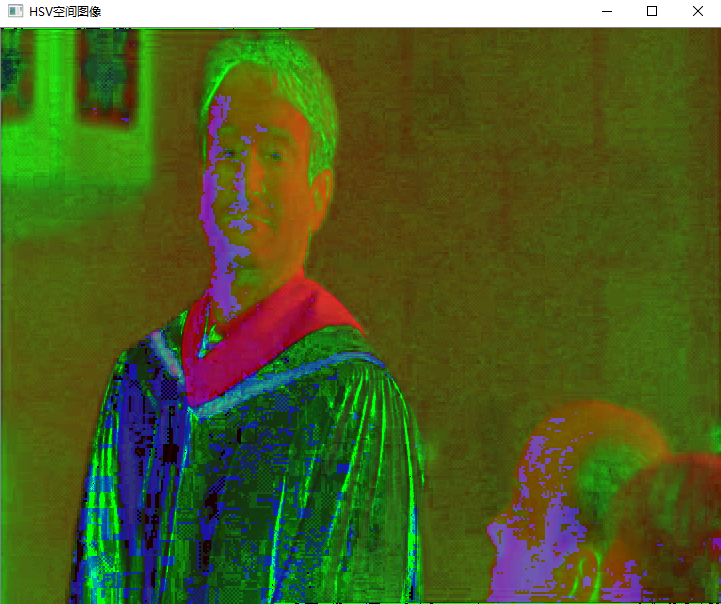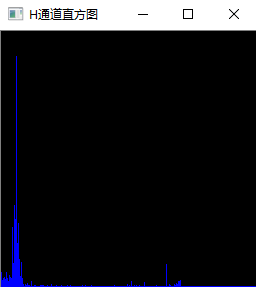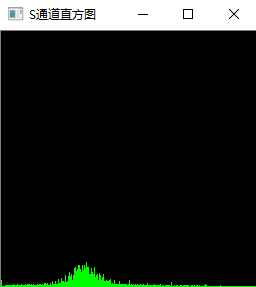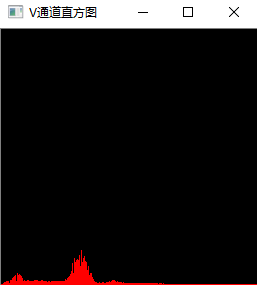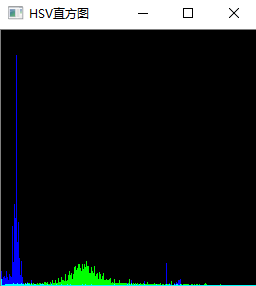## 【数字图像处理杂记】RGB直方图的bins

2018-09-14 13:32:59 gongyi_yf 阅读数 1384
• ###### 利用图像分割和融合技术实现图片滤镜特效

通过本课程学习，初学者可以熟练使用opencv4 API完成各种常见的图像分割、识别任务。 中高级学习者也一定能有新的体会和收获。 本课程所讲的例子代码来自于实际开发项目，有较高的实用性。

20人学习 刘山
免费试看

## 图像处理（三）直方图匹配

2012-10-25 19:38:21 guoyk1990 阅读数 21451
• ###### 利用图像分割和融合技术实现图片滤镜特效

通过本课程学习，初学者可以熟练使用opencv4 API完成各种常见的图像分割、识别任务。 中高级学习者也一定能有新的体会和收获。 本课程所讲的例子代码来自于实际开发项目，有较高的实用性。

20人学习 刘山
免费试看

直方图匹配，又称直方图规定化，即变换原图的直方图为规定的某种形式的直方图，从而使两幅图像具有类似的色调和反差。直方图匹配属于非线性点运算。

直方图规定化的原理：对两个直方图都做均衡化，变成相同的归一化的均匀直方图，以此均匀直方图为媒介，再对参考图像做均衡化的逆运算

以下是算法实现（C#）

    /// <summary>
/// 直方图匹配
/// </summary>
/// <param name="srcBmp">原始图像</param>
/// <param name="matchingBmp">匹配图像</param>
/// <param name="dstBmp">处理后图像</param>
/// <returns>处理成功 true 失败 false</returns>
public static bool HistogramMatching(Bitmap srcBmp, Bitmap matchingBmp, out Bitmap dstBmp) {
if (srcBmp == null || matchingBmp == null) {
dstBmp = null;
return false;
}
dstBmp = new Bitmap(srcBmp);
Bitmap tempSrcBmp = new Bitmap(srcBmp);
Bitmap tempMatchingBmp = new Bitmap(matchingBmp);
double[] srcCpR = null;
double[] srcCpG = null;
double[] srcCpB = null;
double[] matchCpB = null;
double[] matchCpG = null;
double[] matchCpR = null;
//分别计算两幅图像的累计概率分布
getCumulativeProbabilityRGB(tempSrcBmp, out srcCpR, out srcCpG, out srcCpB);
getCumulativeProbabilityRGB(tempMatchingBmp, out matchCpR, out matchCpG, out matchCpB);

double diffAR = 0, diffBR = 0, diffAG = 0, diffBG = 0, diffAB = 0, diffBB = 0;
byte kR = 0, kG = 0, kB = 0;
//逆映射函数
byte[] mapPixelR = new byte;
byte[] mapPixelG = new byte;
byte[] mapPixelB = new byte;
//分别计算RGB三个分量的逆映射函数
//R
for (int i = 0; i < 256; i++) {
diffBR = 1;
for (int j = kR; j < 256; j++) {
//找到两个累计分布函数中最相似的位置
diffAR = Math.Abs(srcCpR[i] - matchCpR[j]);
if (diffAR - diffBR < 1.0E-08) {//当两概率之差小于0.000000001时可近似认为相等
diffBR = diffAR;
//记录下此时的灰度级
kR = (byte)j;
}
else {
kR = (byte)Math.Abs(j - 1);
break;
}
}
if (kR == 255) {
for (int l = i; l < 256; l++) {
mapPixelR[l] = kR;
}
break;
}
mapPixelR[i] = kR;
}
//G
for (int i = 0; i < 256; i++) {
diffBG = 1;
for (int j = kG; j < 256; j++) {
diffAG = Math.Abs(srcCpG[i] - matchCpG[j]);
if (diffAG - diffBG < 1.0E-08) {
diffBG = diffAG;
kG = (byte)j;
}
else {
kG = (byte)Math.Abs(j - 1);
break;
}
}
if (kG == 255) {
for (int l = i; l < 256; l++) {
mapPixelG[l] = kG;
}
break;
}
mapPixelG[i] = kG;
}
//B
for (int i = 0; i < 256; i++) {
diffBB = 1;
for (int j = kB; j < 256; j++) {
diffAB = Math.Abs(srcCpB[i] - matchCpB[j]);
if (diffAB - diffBB < 1.0E-08) {
diffBB = diffAB;
kB = (byte)j;
}
else {
kB = (byte)Math.Abs(j - 1);
break;
}
}
if (kB == 255) {
for (int l = i; l < 256; l++) {
mapPixelB[l] = kB;
}
break;
}
mapPixelB[i] = kB;
}
//映射变换
BitmapData bmpData = dstBmp.LockBits(new Rectangle(0, 0, dstBmp.Width, dstBmp.Height), ImageLockMode.ReadWrite, PixelFormat.Format24bppRgb);
unsafe {
byte* ptr = null;
for (int i = 0; i < dstBmp.Height; i++) {
ptr = (byte*)bmpData.Scan0 + i * bmpData.Stride;
for (int j = 0; j < dstBmp.Width; j++) {
ptr[j * 3 + 2] = mapPixelR[ptr[j * 3 + 2]];
ptr[j * 3 + 1] = mapPixelG[ptr[j * 3 + 1]];
ptr[j * 3] = mapPixelB[ptr[j * 3]];
}
}
}
dstBmp.UnlockBits(bmpData);
return true;
}

/// <summary>
/// 计算各个图像分量的累计概率分布
/// </summary>
/// <param name="srcBmp">原始图像</param>
/// <param name="cpR">R分量累计概率分布</param>
/// <param name="cpG">G分量累计概率分布</param>
/// <param name="cpB">B分量累计概率分布</param>
private static void getCumulativeProbabilityRGB(Bitmap srcBmp, out double[] cpR, out double[] cpG, out double[] cpB) {
if (srcBmp == null) {
cpB = cpG = cpR = null;
return;
}
cpR = new double;
cpG = new double;
cpB = new double;
int[] hR = null;
int[] hG = null;
int[] hB = null;
double[] tempR = new double;
double[] tempG = new double;
double[] tempB = new double;
getHistogramRGB(srcBmp, out hR, out hG, out hB);
int totalPxl = srcBmp.Width * srcBmp.Height;
for (int i = 0; i < 256; i++) {
if (i != 0) {
tempR[i] = tempR[i - 1] + hR[i];
tempG[i] = tempG[i - 1] + hG[i];
tempB[i] = tempB[i - 1] + hB[i];
}
else {
tempR = hR;
tempG = hG;
tempB = hB;
}
cpR[i] = (tempR[i] / totalPxl);
cpG[i] = (tempG[i] / totalPxl);
cpB[i] = (tempB[i] / totalPxl);
}
}

    /// <summary>
/// 获取图像三个分量的直方图数据
/// </summary>
/// <param name="srcBmp">图像</param>
/// <param name="hR">R分量直方图数据</param>
/// <param name="hG">G分量直方图数据</param>
/// <param name="hB">B分量直方图数据</param>
public static void getHistogramRGB(Bitmap srcBmp, out int[] hR, out int[] hG, out int[] hB) {
if (srcBmp == null) {
hR = hB = hG = null;
return;
}
hR = new int;
hB = new int;
hG = new int;
BitmapData bmpData = srcBmp.LockBits(new Rectangle(0, 0, srcBmp.Width, srcBmp.Height), ImageLockMode.ReadOnly, PixelFormat.Format24bppRgb);
unsafe {
byte* ptr = null;
for (int i = 0; i < srcBmp.Height; i++) {
ptr = (byte*)bmpData.Scan0 + i * bmpData.Stride;
for (int j = 0; j < srcBmp.Width; j++) {
hB[ptr[j * 3]]++;
hG[ptr[j * 3 + 1]]++;
hR[ptr[j * 3 + 2]]++;
}
}
}
srcBmp.UnlockBits(bmpData);
return;
}## 数字图像处理实验（1）——直方图均衡化

2020-04-07 12:53:39 qq_41650371 阅读数 386
• ###### 利用图像分割和融合技术实现图片滤镜特效

通过本课程学习，初学者可以熟练使用opencv4 API完成各种常见的图像分割、识别任务。 中高级学习者也一定能有新的体会和收获。 本课程所讲的例子代码来自于实际开发项目，有较高的实用性。

20人学习 刘山
免费试看

## 源码：

// 灰度图像
clear
%读入彩色图像将其灰度化
imshow(pic)		%显示出来
title('输入的灰色png图像')

%绘制直方图
[m,n]=size(pic);	%测量图像尺寸参数
GP=zeros(1,256);	%预创建存放灰度出现概率的向量
N=zeros(1,256);
for k=0:255
N(k+1) = length(find(pic==k));
GP(k+1)=length(find(pic==k))/(m*n); 	% 计算每级灰度出现的概率，将其存入 GP 中相应位置
end
figure,bar(0:255,GP,'b')	%绘制直方图title('原图像直方图')
xlabel('灰度值')
ylabel('出现概率')

%直方图均衡化
CF=zeros(1,256);
for i=1:256
for j=1:i
CF(i)=GP(j)+CF(i);	%计算 Sk
end
end
S2=round((CF*256)+0.5);	%将 Sk 归到相近级的灰度
for i=1:256
GPeq(i)=sum(GP(find(S2==i)));	%计算现有每个灰度级出现的概率
end
figure,bar(0:255,GPeq,'b')	%显示均衡化后的直方图title('均衡化后的直方图')
xlabel('灰度值')
ylabel('出现概率')

%图像均衡化
PA=pic;
for i=0:255
PA(find(pic==i))=S2(i+1);	%将各个像素归一化后的灰度值赋给这个像素
end
figure,imshow(PA)

%绘制灰度级变换曲线
figure
plot(0:255,S2,'r')
xlabel('均值化前')
ylabel('均值化后')
grid on
legend('灰度级变换曲线');


// 彩色图像
function pic2()
clear;
imshow(pic)		%显示出来
title('原图像');

[M,N,D] = size(pic);
%提取单通道分量
pic=double(pic);
r=pic(:,:,1);
g=pic(:,:,2);
b=pic(:,:,3);
%实现转换
angle=acos(0.5*((r-g)+(r-b))./(sqrt((r-g).^2+(r-b).*(g-b))));
if (b>=g)
H = 2*pi-angle;
else
H = angle;
end
H=H/(2*pi);
S=1-3.*(min(min(r,g),b))./(r+g+b);
H(S==0)=0;
I=(r+g+b)/3;
I=uint8(I);%范围不超[0,255]
%统计像素亮度
INumPixel = zeros(1,256);%用长度为256的一维数组统计各灰度值的数目
for i = 1:M
for j = 1: N
INumPixel(I(i,j) + 1) = INumPixel(I(i,j) + 1) + 1;
end
end
%亮度直方图
GP = zeros(1,256);
for i = 1:256
GP(i) = INumPixel(i) / (M * N * 1.0);
end
figure,bar(0:255,GP,'b')	%绘制直方图title('原图像直方图')
xlabel('亮度值')
ylabel('出现概率')

%直方图均衡化
S2 = zeros(1,256);
for i = 1:256
if i == 1
S2(i) = GP(i);
else
S2(i) = S2(i - 1) + GP(i);
end
end
%将S2乘上最大灰度255并且向上取整
S2 = uint8(255 .* S2 + 0.5);
%将原图像各个位置灰度值映射到新值
for i = 1:M
for j = 1: N
Inew(i,j) = S2(I(i,j)+1);
end
end
for i=1:256
GPeq(i)=sum(GP(find(S2==i)));	%计算现有每个灰度级出现的概率
end
figure
plot(0:255,GPeq,'b')	%显示均衡化后的直方图title('均衡化后的直方图')
xlabel('亮度值')
ylabel('出现概率')

%绘制亮度级变换曲线
figure
plot(0:1:255,S2,'r')
xlabel('均值化前')
ylabel('均值化后')
grid on
legend('亮度级变换曲线');

I=Inew;
H=H*2*pi;
I=double(I);
S=double(S);
H=double(H);
[m,n]=size(H);
%转换
for i = 1:m
for j = 1:n
if (0<=H(i,j))&(H(i,j)<2*pi/3)
B(i,j)=I(i,j).*(1-S(i,j));
R(i,j)=I(i,j).*(1+S(i,j).*cos(H(i,j))./cos(pi/3-H(i,j)));
G(i,j)=3*I(i,j)-(R(i,j)+B(i,j));
end
if (2*pi/3<=H(i,j))&(H(i,j)<4*pi/3)
H(i,j)=H(i,j)-2*pi/3;
R(i,j)=I(i,j).*(1-S(i,j));
G(i,j)=I(i,j).*(1+S(i,j).*cos(H(i,j)-2*pi/3)./cos(pi-H(i,j)));
B(i,j)=3*I(i,j)-(R(i,j)+G(i,j));
end
if (4*pi/3<=H(i,j))& (H(i,j)<2*pi)
H(i,j)=H(i,j)-4*pi/3;
G(i,j)=I(i,j).*(1-S(i,j));
B(i,j)=I(i,j).*(1+S(i,j).*cos(H(i,j)-4*pi/3)./cos(5*pi/3-H(i,j)));
R(i,j)=3*I(i,j)-(G(i,j)+B(i,j));
end
end
end

output3=cat(3,R,G,B);
output3=uint8(output3);
figure
imshow(output3)


## 实验结果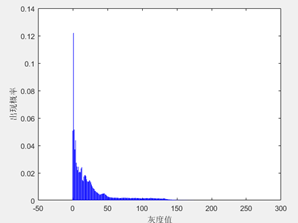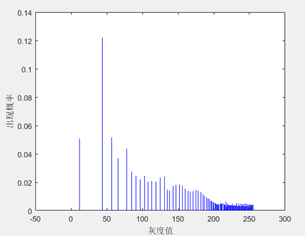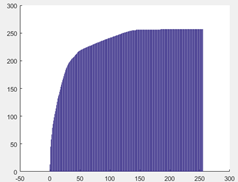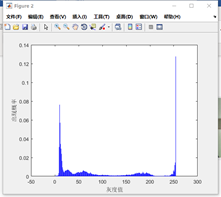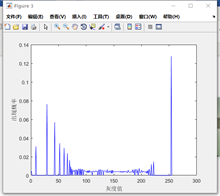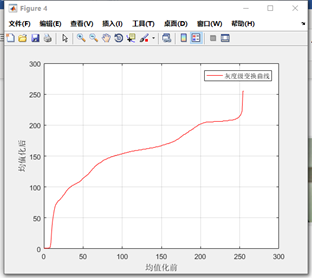## 图像处理大型科普——图像直方图

2016-05-07 18:16:58 qq404752007 阅读数 7384
• ###### 利用图像分割和融合技术实现图片滤镜特效

通过本课程学习，初学者可以熟练使用opencv4 API完成各种常见的图像分割、识别任务。 中高级学习者也一定能有新的体会和收获。 本课程所讲的例子代码来自于实际开发项目，有较高的实用性。

20人学习 刘山
免费试看

# 图像处理大型科普——图像直方图

## 分不清的先去一旁罚歌一首## 继续说正事## 前方高能，集中注意a和b是两张图的轮廓点集，c是一个坐标系，把一个圆按照离圆心的距离、和正北的角度两个指标分成60个块（角度分成12类区域，距离分成5类区域，12*5=60），然后遍历点集的每一个点，以当前遍历的点为C坐标的圆心，把其他点按60个区域划分，变成一个size = 60的素组，保存着每个块中的轮廓点数量，现在每个点都对应了一个关于其他点的直方图，就像d、e、f三张图一样，60个小方块，点的数量越多，颜色越深。